Get instant live expert help with Excel or Google Sheets“My Excelchat expert helped me in less than 20 minutes, saving me what would have been 5 hours of work!”

#### Post your problem and you’ll get Expert help in seconds.

Your message must be at least 40 characters
Our professional Expert are available now. Your privacy is guaranteed.

# Normalize size units to GigabytesRead time: 30 minutes

A byte is a unit of memory size and the decimal equivalent of one gigabyte is 1,000,000 bytes.  Other units of computer storage and memory include kilobytes, megabytes, terabytes, petabytes, and many more.   This step by step tutorial will assist all levels of Excel users in normalizing size units into gigabytes.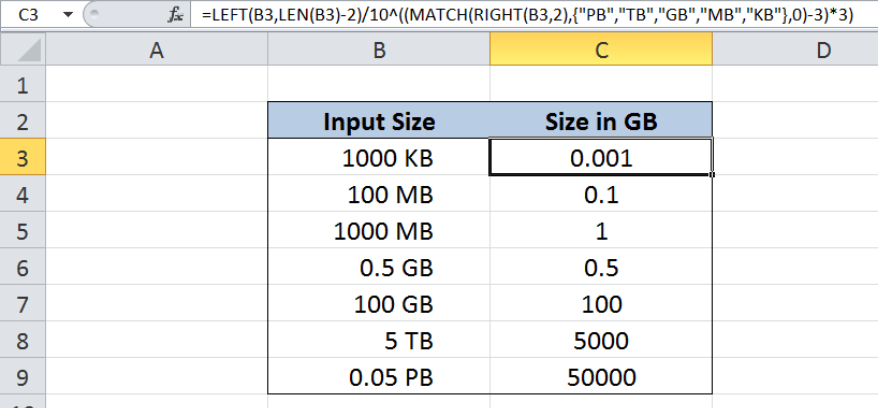Figure 1.  Final result:  Normalize size units to gigabytes

Final formula: `=LEFT(B3,LEN(B3)-2)/10^((MATCH(RIGHT(B3,2),{"PB","TB","GB","MB","KB"},0)-3)*3)`

## Syntax of LEFT and RIGHT functions

LEFT extracts a substring from the left side of a text string

`=LEFT (text,[num_chars])`

RIGHT extracts a substring from the right side of a text string

`=RIGHT(text,[num_chars])`

• text – the text string containing the characters or substring we want to extract
• num_chars   Optional; determines the number of characters we want to extract

## Syntax of LEN function

LEN returns the length of a text string in number of characters

`=LEN(text)`

• text  – the text whose length we want to determine. Spaces are counted as characters

## Syntax of MATCH function

MATCH function returns the position of a value in a range

`=MATCH(lookup_value, lookup_array, [match_type])`

• lookup_value – a value which we want to find in the lookup_array
• lookup_array – the range of cells containing the value we want to match
• [match_type] optional; the type of match; if omitted, the default value is 1; We use 0 to find an exact match

## Setting up our Data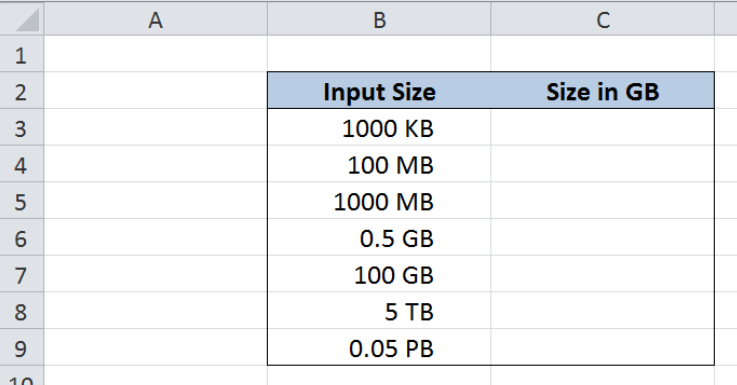Figure 2.  Sample data to normalize size units to gigabytes

Our table consists of two columns: Input Size (column B) and Size in GB (column C).  Our data in column B contains storage and memory values in different units.

We want to convert the values in column B and normalize them into gigabytes “GB”, regardless of the original units.  For our formula to work, the values in column B must contain the unit in the last two characters of the string. The results will be recorded in column C.

We will use below table as our guide in the conversion of units, where KB, MB, GB, TB and PB are represented in decimal multiples of bytes.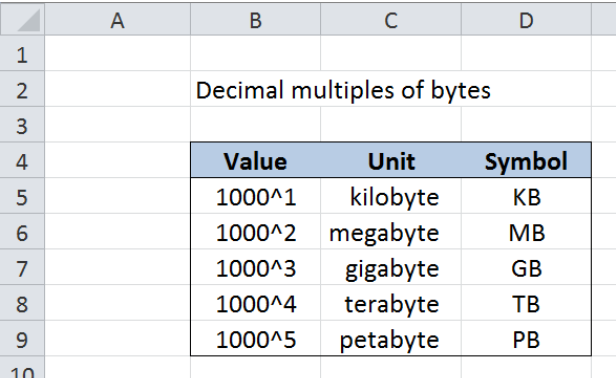Figure 3.  Decimal multiples of bytes

## Normalize size units to gigabytes

We want to be able to convert all values in column B into one uniform unit which is GB (gigabytes).  We can do this by using a combination of the LEFT, LEN, RIGHT and MATCH functions. Let us follow these steps:

Step 1.  Select cell C3

Step 2.  Enter the formula: `=LEFT(B3,LEN(B3)-2)/10^((MATCH(RIGHT(B3,2),{"PB","TB","GB","MB","KB"},0)-3)*3)`

Step 3.  Press ENTER

Step 4.  Copy the formula in C3 to cells C4:C9 by clicking the “+” icon at the bottom-right corner of cell C3 and dragging it down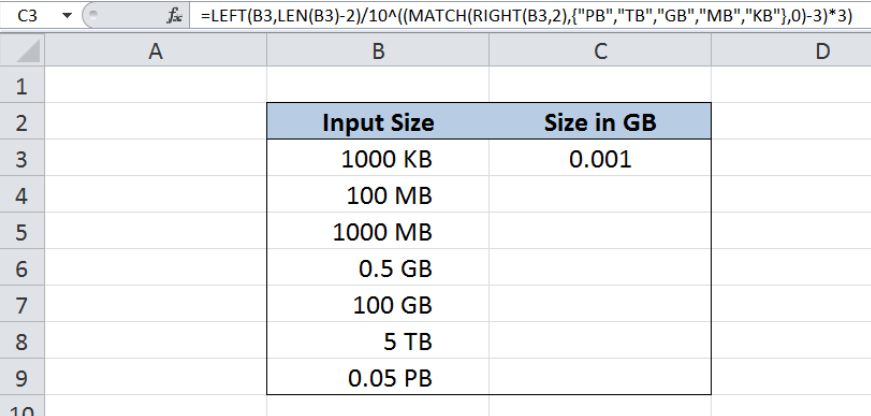Figure 4.  Entering the formula to normalize size units to gigabytes

Our formula extracts the numeric value of the size in column B, then divides it by the corresponding divisor to normalize the given unit into gigabytes.

The formula has two main components,

• `LEFT(B3,LEN(B3)-2)`  extracts the numeric value of the size in column B
• In order to get the numeric value, the formula extracts the characters from the left of B3, but not including the last 2 characters that represent the unit.
• `10^((MATCH(RIGHT(B3,2),{"PB","TB","GB","MB","KB"},0)-3)*3) `divisor that converts the unit into gigabytes, represented as a power of 10
• First, the formula matches the unit in B3 to the array {“PB”,”TB”,”GB”,”MB”,”KB”}
• In the first example, the unit is KB so MATCH returns the value 5, since KB is the fifth in the list
• The formula becomes 10^((5-3)*3) or 10^6, which is correct because there are 10^6 KB in 1 GB

Finally, cell C3 is calculated as 1000/10^6 or 0.001.

For the next two examples bearing the unit “MB”, the MATCH function returns the value 4, making the divisor 10^((4-3)*3 or 10^3.  This corresponds to the conversion factor of 10^3 MB in 1 GB. As a result, the values in cells C4 and C5 are 0.1 and 1, respectively.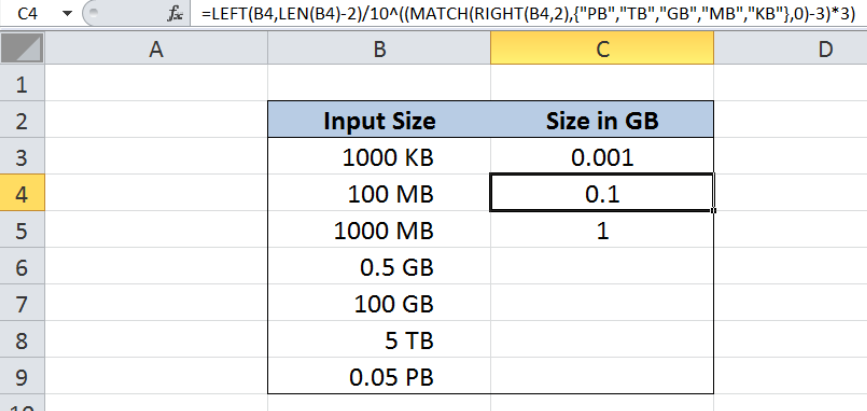Figure 5.  Converting megabytes to gigabytes

The values in cells B6 and B7 are already in GB, so the values in column C are the same and no conversion is needed.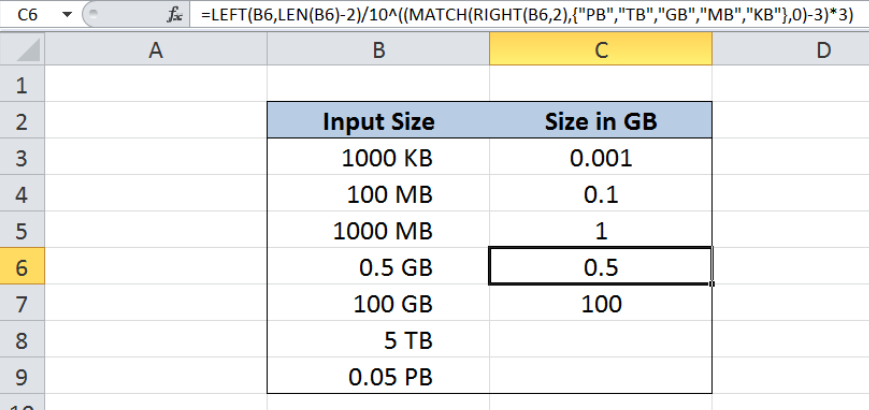Figure 6.  Formula returns same values in gigabytes

Below table shows the final results in normalizing size units to gigabytes by using the LEFT, LEN, RIGHT and MATCH functions in Excel.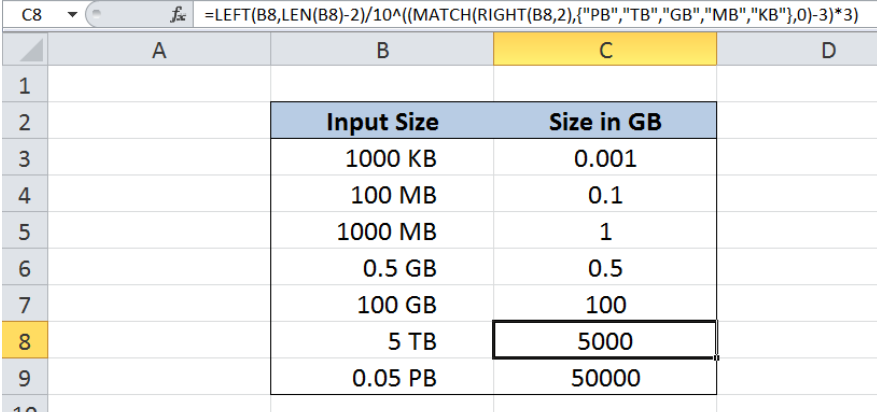Figure 7.  Output: Normalize size units to gigabytes

Most of the time, the problem you will need to solve will be more complex than a simple application of a formula or function. If you want to save hours of research and frustration, try our live Excelchat service! Our Excel Experts are available 24/7 to answer any Excel question you may have. We guarantee a connection within 30 seconds and a customized solution within 20 minutes.

### Did this post not answer your question? Get a solution from connecting with the expert.Another blog reader asked this question today on Excelchat:
Solution examplesWhat is the formula for splitting up one column text into 2 columns?
Solved by B. H. in 20 minsI have text and number in multiple cells (Ex: Cell 1: 4S, Cell 2: 3.5A, Cell 3: 8O) and want to sum the number values only (Ex: 4 + 3.5 + 8). How can I do this?
Solved by O. A. in 60 minsI need help with a vlookup type formula that will take a given name and reference it against a list of names in columns D-O. then take a given date and reference it against dates in columns A10-A440, then return the cell or its contents at the intersection of those that column and row.
Solved by I. H. in 13 minsI am having issues creating the one way data table to show the means and standard deviations when you change the proportion of portfolio 1. I have the mean formula and the standard deviation formula at the top and -1 through 1 down the left side. I highlight all of it and create a data table. I reference the "proportion of portfolio 1" cell in the column input box but when the data table comes out it has all the same numbers all they way down the table.
Solved by G. Q. in 49 minsI need to create a formula that extracts the prefix from column A
Solved by I. Q. in 42 mins## Subscribe to Excelchat.coAnother blog reader asked this question today on Excelchat: